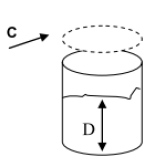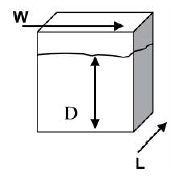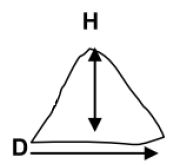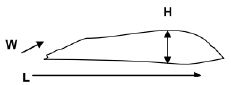## Hopper Bottom Bin

Use the conical pile formula for the hopper and the round bin formula for the upper portion of the bin. Combine the results of both formulas for the total volume of grain in the bin.

## Round BinC = Distance around bin
D = Depth of the grain

### Formula

C x C x D x 0.07958 = Volume

Imperial Measure – Feet
C = is 32’ around the bin
D = is 12’ depth of grain
32’ x 32’ x 12’ x 0.07958 = 977.9 ft3
977.9 x 0 .7786 = 761 bushels

Metric Measure – Meters
C = is 9.8 meters
D = is 3.7 meters of grain
9.8m x 9.8m x 3.7m x 0.07958 = 28.2 m3
28.2 m3 / 0.036 = 777 bushels

## Square or Rectangular BinL = Length of bin’s side
W = Width
D = Depth of grain

### Formula

L x W x D = Volume

Imperial Measure – Feet
L = 10’ long
W = 8’ wide
D = 12’ of grain
10’ x 8’ x 12’ = 960 ft3
960 x 0.7786 = 747.5 bushels

Metric Measure – Meters
L = 3.0 meters long
W = 2.4 meters wide
D = 3.7 meters of grain
3.0m x 2.4m x 3.7m = 26.6 m3
26.6 m3 / 0.036 = 750 bushels

## Conical Pile of GrainD = Diameter
H = Height of the grain

### Formula

D x D x H x 0.31416 = Volume

Imperial Measure – Feet
D = 14’ diameter
H = 9’ high pile of grain
If the pile is lopsided you may need to measure in at least two places across the base and determine an average diameter
14’ x 14’ x 9’ x 0.31416 = 554.2 ft3
554.8 x 0.7786 = 431.5 bushels

Metric Measure – Meters
D = 4.3 meters diameter
H = 2.7 meters high
4.3m x 4.3m x 2.7m x 0.31416 = 15.7 m3
15.7 m3 / 0.036 = 436 bushels

## Triangular Pile of GrainL = Length of the pile
W = Width of the pile
H = Height of the grain

### Formula

L x W x H x 0.5 = Volume

Imperial Measure – Feet
L = 25’ long pile
W = 15’ across the pile
H = 7’ height of grain pile
25’ x 15’ x 7’ x 0.5 = 1312.5 ft3
1312.5 x 0.7786 = 1021.9 bushels

Metric Measure – Meters
L = 7.6m long pile
W = 4.7m across the pile
H = 2.1m height of grain
7.6m x 4.7m x 2.1m x 0.5 = 37.5 m3
37.5 m3 / 0.036 = 1041 bushels

Things to Note:

• Differences between metric and imperial calculations are due to rounding.
• When grain is difficult to measure, production may not be determined and indemnities may not be calculated until grain is sold or in a measurable position.